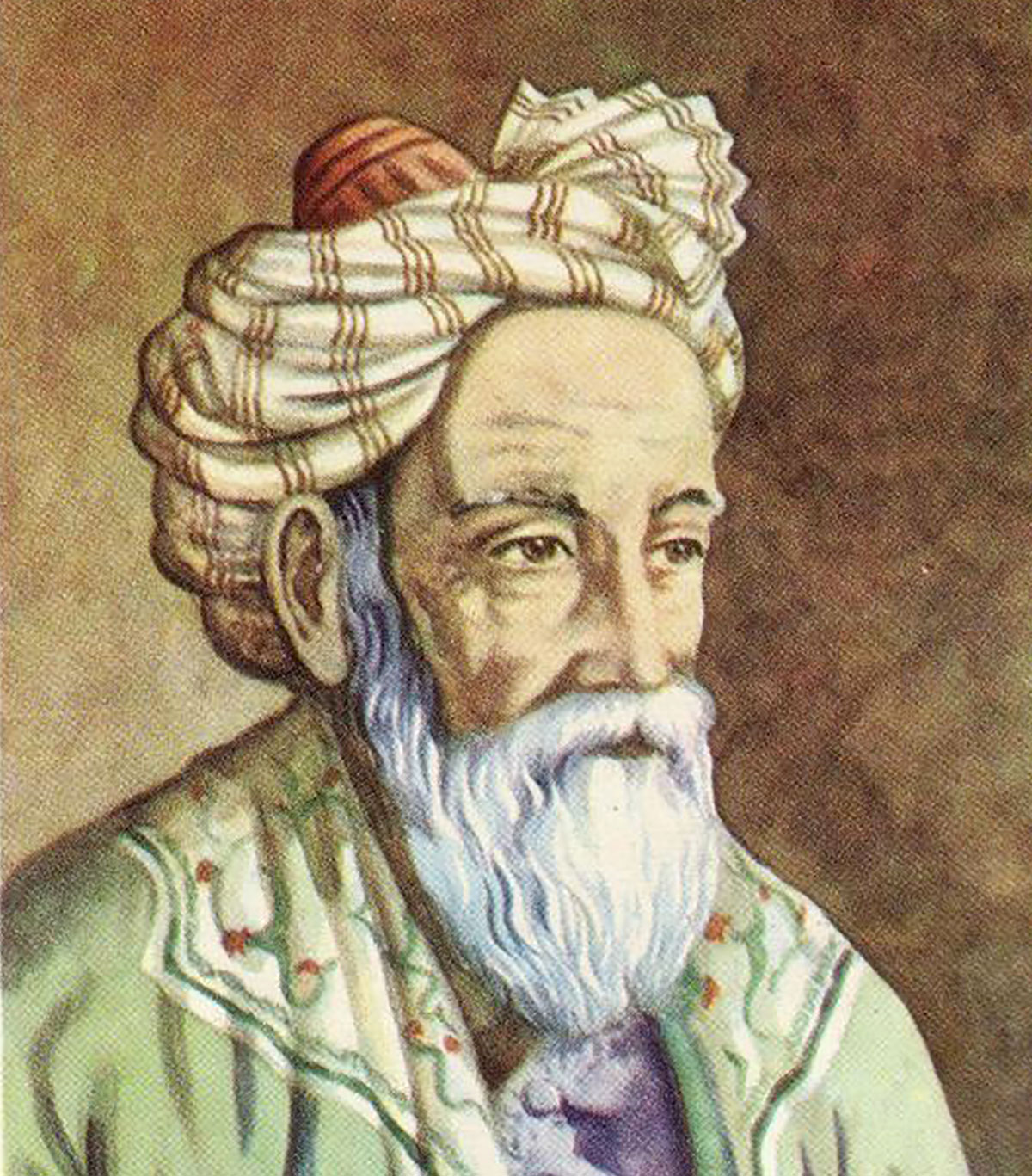In simple terms, we observe mathematics as the study of numbers, quantities, structures, spaces and variations, which involves the use of certain patterns and some logic to test the truth or falsity of statements or some hypothesis.

The mathematics has evolved from basic counting, measurements, to the study of dynamics and to various scientific, medical, financial and engineering orientated peaks of influence.

The world-renowned Italian scientist Galileo Galilei was of the opinion that we can’t interpret our universe until we can’t interpret the language in which it has been written down. Of course, it is the language of mathematics, the language of counting, the language of lines, triangles, circles, polygons and various mathematical structures.Omar Khayyam

The history of mathematics has seen the influence of various notable mathematicians whose work made the foundational and remarkable importance in the subject. Around 3000 BC, the Babylonians and Egyptians started use of arithmetic, algebra, and geometry for calculations, construction and astronomy. In the sixth century BC, the Greeks made a systematic study of mathematics. In the 300 BC, Euclid introduced the axiomatic method even used today. His writings Elements is regarded as the most successful and influential textbook of all time. The ancient Greek mathematician Archimedes is observed as one of the leading mathematician ever born. The ancient Greeks worked in the field of conics, trigonometry and algebra.

The Hindu-Arabic numeral system, a positional number system, which is the most common system for the symbolic representation of numbers in the world, was invented in India between first and fourth century. It was later transmitted to the West through Islamic world.

The most notable development was observed in the golden age of Islam during ninth and tenth century in the form of development of algebra by a Persian mathematician named Muhammad ibn Musa all Khawarizimi.

The Islamic world advanced in the study of trigonometry and addition of a decimal point to Arabic numeral system by notable work of Omer Khayam and Sharaf al-din al Tusi.

The independent development of modern calculus by Issac Newton and Leibniz revolutionised the mathematical world. Meanwhile, Leonhard Euler also became influential by introducing Complexes as the largest possible number system and with the help of his numerous theorems and discoveries (like a solution to historical Konigsberg bridge problem).

In fact, Carl Friedrich Gauss emerged as one of the foremost mathematicians of the nineteenth century who contributed in the fields of analysis, geometry, algebra, and number theory.

The extension of boundaries of mathematics, solutions to problems, conjectures, and the introduction of new concepts continued in twentieth and twenty-first century maintaining the leading position and importance of mathematics in regards to various disciplines of knowledge.

Instead of all the importance and such interesting historical features in support, we have greatly failed in providing or creating a beautiful mathematical environment from schools to universities. That is because, instead of some achievement or world-class research in the subject, we have produced a number of students who either observe mathematics as a boring subject or fear mathematics because they fail in getting the various formal techniques which are used for evaluating various mathematical problems.

The credit of ruining the beauty of mathematics or credit of making mathematics a less interesting subject goes from a classroom tutor to various academics caring systems.

A teacher can build confidence and motivate a student in doing mathematics and introduce it as a useful and interesting tool for a student. Later a student can improve his/her basic skills, which will lead to some logical and grownup minds aimed at solving various social orientated problems and designing some mathematical models.

The tricky and skilful mathematics can be taught at elementary levels. The global mathematical exposure is required in making mathematics a subject of interest or desire for the readers.

(A resident of Chairhara, Magam (Budgam), the author is pursuing MSc mathematics at the Central University of Kashmir.)